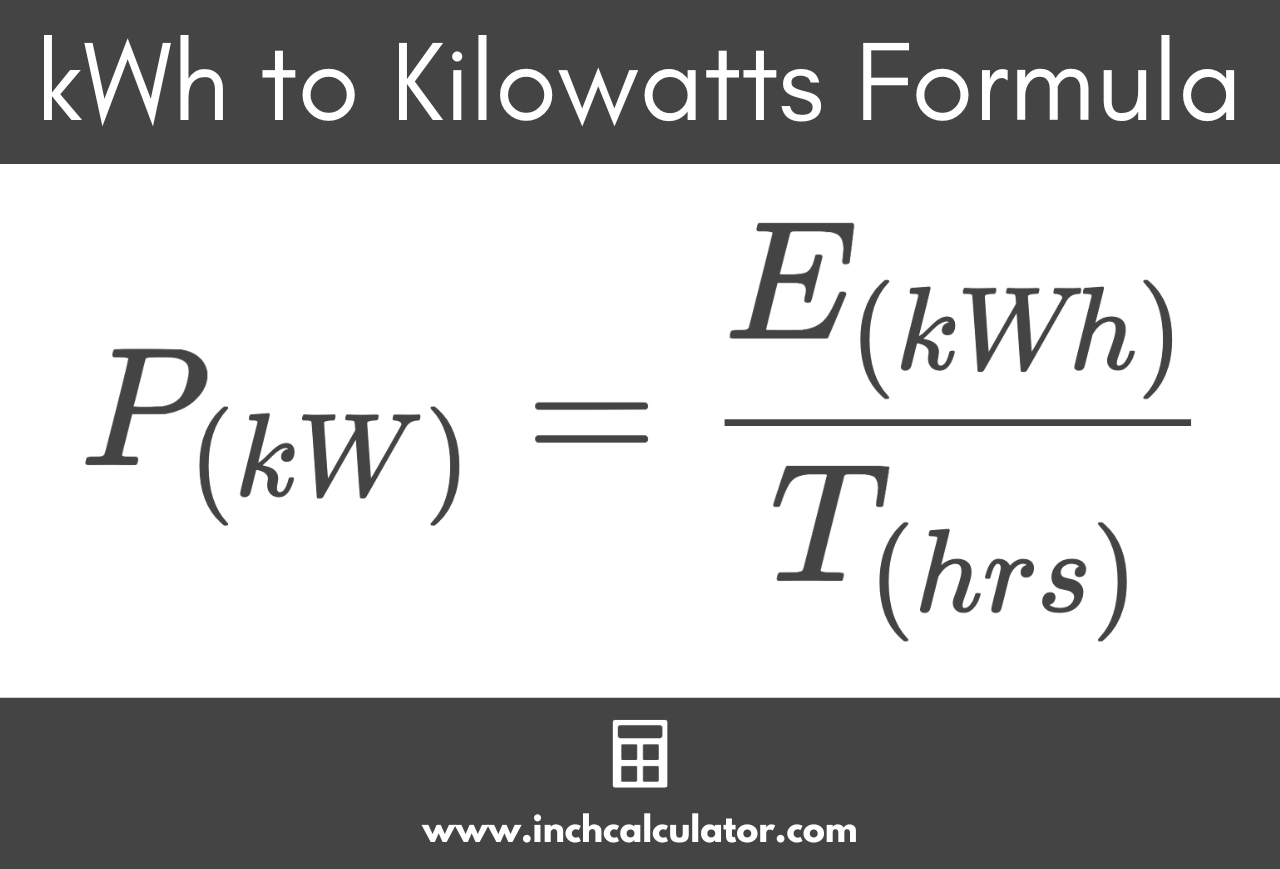# Kilowatt-Hours (kWh) to Kilowatts (kW) Conversion Calculator

Convert energy in kilowatt-hours to power in kilowatts by entering the energy (in kWh) and time (in hours) below.

## Power in Kilowatts:

kW
Learn how we calculated this below

## How to Convert Kilowatt-Hours to Kilowatts

Kilowatt-hours, expressed kWh or kW·h, are a measure of electrical energy consumption equivalent to one kilowatt of power used for one hour. The electric company uses this measurement to calculate how much to bill customers, for example.

Kilowatts, abbreviated kW, are a measure of electric power.

### kWh to kW Conversion Formula

To convert energy in kWh to power in kW, use the formula below.

P(kW) = E(kWh) / T(hrs)

So, the power P in kilowatts is equal to the energy E in kilowatt-hours divided by the time period T measured in hours.For example, let’s convert 48 kWh of energy consumption over a 24-hour time period to kW.

kW = 48 kWh ÷ 24 hours
kW = 2 kW

Do you want to convert kW to kWh?

## kWh to kW Conversion Table

Table showing the power in kilowatts converted to the energy in kilowatt-hours for various lengths of time.
Energy (in kWh) Time (in hours) Power (in kW)
100 kWh 1 hr 100 kW
100 kWh 2 hrs 50 kW
100 kWh 3 hrs 33.33 kW
100 kWh 4 hrs 25 kW
100 kWh 5 hrs 20 kW
100 kWh 6 hrs 16.67 kW
100 kWh 7 hrs 14.29 kW
100 kWh 8 hrs 12.5 kW
100 kWh 9 hrs 11.11 kW
100 kWh 10 Hrs 10 kW
100 kWh 15 hrs 6.67 kW
100 kWh 20 hrs 5 kW
100 kWh 25 hrs 4 kW
100 kWh 30 hrs 3.33 kW
100 kWh 35 hrs 2.86 kW
100 kWh 40 hrs 2.5 kW
100 kWh 45 hrs 2.22 kW
100 kWh 50 hrs 2 kW
100 kWh 55 hrs 1.82 kW
100 kWh 60 hrs 1.67 kW
100 kWh 65 hrs 1.54 kW
100 kWh 70 hrs 1.43 kW
100 kWh 75 hrs 1.33 kW
100 kWh 80 hrs 1.25 kW
100 kWh 85 hrs 1.18 kW
100 kWh 90 hrs 1.11 kW
100 kWh 95 hrs 1.05 kW
100 kWh 100 hrs 1 kW

You might also be interested in our kWh to watts calculator.